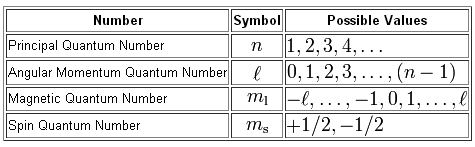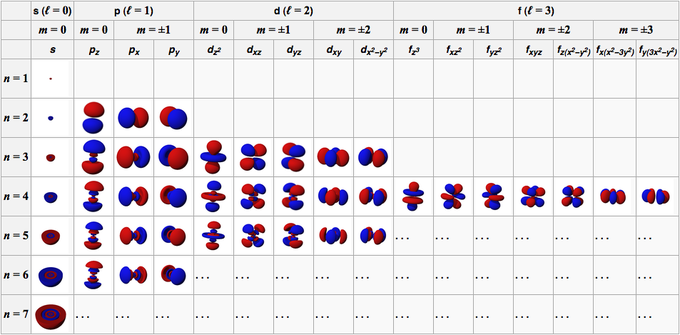## Quantum Numbers

#### Learning Objective

• Assign quantum numbers to electrons, noting their physical meaning

#### Key Points

• To completely describe an electron in an atom, four quantum numbers are needed: energy (n), angular momentum (ℓ), magnetic moment (m), and spin (ms).
• The first quantum number describes the electron shell, or energy level, of an atom. The value of n ranges from 1 to the shell containing the outermost electron of that atom.
• The dynamics of any quantum system are described by a quantum Hamiltonian (H).

#### Terms

• angular momentumThe vector product that describes the rotary inertia of a system about an axis.
• quantumThe smallest possible, and therefore indivisible, unit of a given quantity or quantifiable phenomenon.
• quantum numberOne of certain integers or half-integers that specify the state of a quantum mechanical system (such as an electron in an atom).

While the work of Bohr and de Broglie clearly established that electrons take on different discrete energy levels that are related to the atomic radius, their model was a relatively simplistic spherical view. There was an appreciation that the energy level of an electron was related to the principal quantum number n, however there was no numerical means of classifying additional aspects of an electron’s motion in space, such as its orientation or direction. In three dimensions, the solutions of the Schrödinger equation provided a set of three additional quantum numbers that could be used to describe electron behavior even in more complicated many-electron atoms. This was in contrast to previous work that focused on one-electron atoms such as hydrogen.

The question of how many quantum numbers are needed to describe any given system has no universal answer; for each system, one must find the answer by performing a full analysis of the system. Formally, the dynamics of any quantum system are described by a quantum Hamiltonian (H) applied to the wave equation. There is one quantum number of the system corresponding to the energy—the eigenvalue of the Hamiltonian. There is also one quantum number for each operator (O) that commutes with the Hamiltonian (i.e. satisfies the relation HO = OH). Note that the operators defining the quantum numbers should be independent of each other. Often there is more than one way to choose a set of independent operators; so in different situations, different sets of quantum numbers may be used for the description of the same system.

The most prominent system of nomenclature spawned from the molecular orbital theory of Friedrich Hund and Robert S. Mulliken, which incorporates Bohr energy levels as well as observations about electron spin. This model describes electrons using four quantum numbers: energy (n), angular momentum (ℓ), magnetic moment (m), and spin (ms). It is also the common nomenclature in the classical description of nuclear particle states (e.g. protons and neutrons).Quantum numbersThese four quantum numbers are used to describe the probable location of an electron in an atom.

## The Principal Quantum Number

The first quantum number describes the electron shell, or energy level, of an atom. The value of n ranges from 1 to the shell containing the outermost electron of that atom. For example, in caesium (Cs), the outermost valence electron is in the shell with energy level 6, so an electron in caesium can have an n value from 1 to 6. For particles in a time-independent potential, per the Schrödinger equation, it also labels the nth eigenvalue of Hamiltonian (H) (i.e. the energy E with the contribution due to angular momentum, the term involving J2, left out). This number therefore has a dependence only on the distance between the electron and the nucleus (i.e. the radial coordinate r). The average distance increases with n, thus quantum states with different principal quantum numbers are said to belong to different shells.

## The Azimuthal Quantum Number

The second quantum number, known as the angular or orbital quantum number, describes the subshell and gives the magnitude of the orbital angular momentum through the relation. In chemistry and spectroscopy, ℓ = 0 is called an s orbital, ℓ = 1 a p orbital, ℓ = 2 a d orbital, and ℓ = 3 an f orbital. The value of ℓ ranges from 0 to n − 1 because the first p orbital (ℓ = 1) appears in the second electron shell (n = 2), the first d orbital (ℓ = 2) appears in the third shell (n = 3), and so on. In chemistry, this quantum number is very important since it specifies the shape of an atomic orbital and strongly influences chemical bonds and bond angles.

## The Magnetic Quantum Number

The magnetic quantum number describes the energy levels available within a subshell and yields the projection of the orbital angular momentum along a specified axis. The values of mℓ range from − to ℓ, with integer steps between them. The s subshell (ℓ = 0) contains one orbital, and therefore the mℓ of an electron in an s subshell will always be 0. The p subshell (ℓ = 1) contains three orbitals (in some systems depicted as three “dumbbell-shaped” clouds), so the mℓ of an electron in a p subshell will be −1, 0, or 1. The d subshell (ℓ = 2) contains five orbitals, with m values of −2, −1, 0, 1, and 2. The value of the m quantum number is associated with the orbital orientation.

## The Spin Projection Quantum Number

The fourth quantum number describes the spin (intrinsic angular momentum) of the electron within that orbital and gives the projection of the spin angular momentum (s) along the specified axis. Analogously, the values of ms range from −s to s, where s is the spin quantum number, an intrinsic property of particles. An electron has spin s = ½, consequently ms will be ±, corresponding with spin and opposite spin. Each electron in any individual orbital must have different spins because of the Pauli exclusion principle, therefore an orbital never contains more than two electrons.

For example, the quantum numbers of electrons from a magnesium atom are listed below. Remember that each list of numbers corresponds to (n, l, ml, ms).

Two s electrons: (1, 0, 0, +½) (1, 0, 0, -½)

Two s electrons: (2, 0, 0, +½) (2, 0, 0, -½)

Six p electrons: (2, 1, -1, +½) (2, 1, -1, -½) (2, 1, 0, +½) (2, 1, 0, -½) (2, 1, 1, +½) (2, 1, 1, -½)

Two s electrons: (3, 0, 0, +½) (3, 0, 0, -½)Table relating quantum numbers to orbital shapeThe relationship between three of the four quantum numbers to the orbital shape of simple electronic configuration atoms up through radium (Ra, atomic number 88). The fourth quantum number, the spin, is a property of individual electrons within a particular orbital. Each orbital may hold up to two electrons with opposite spin directions.